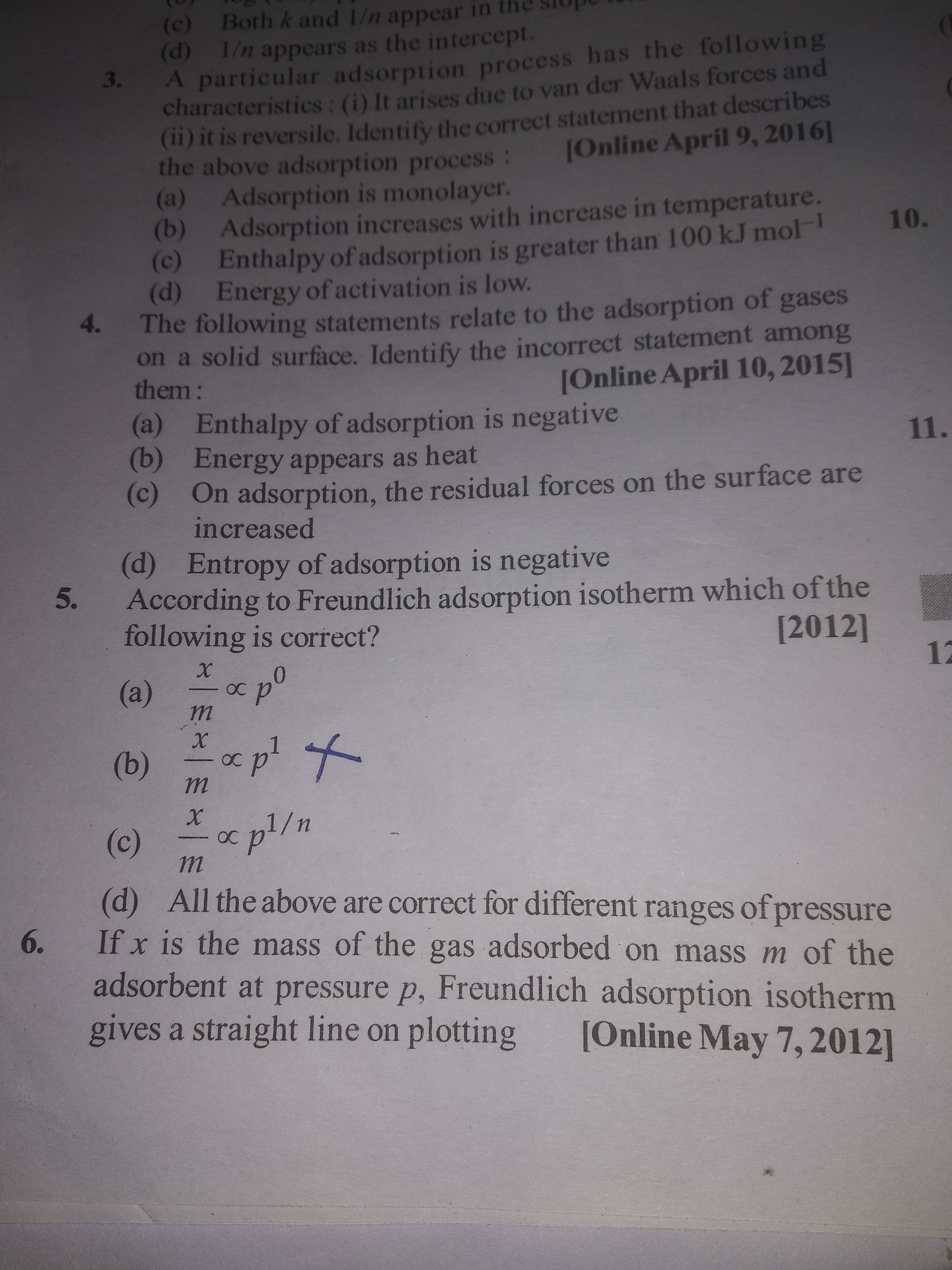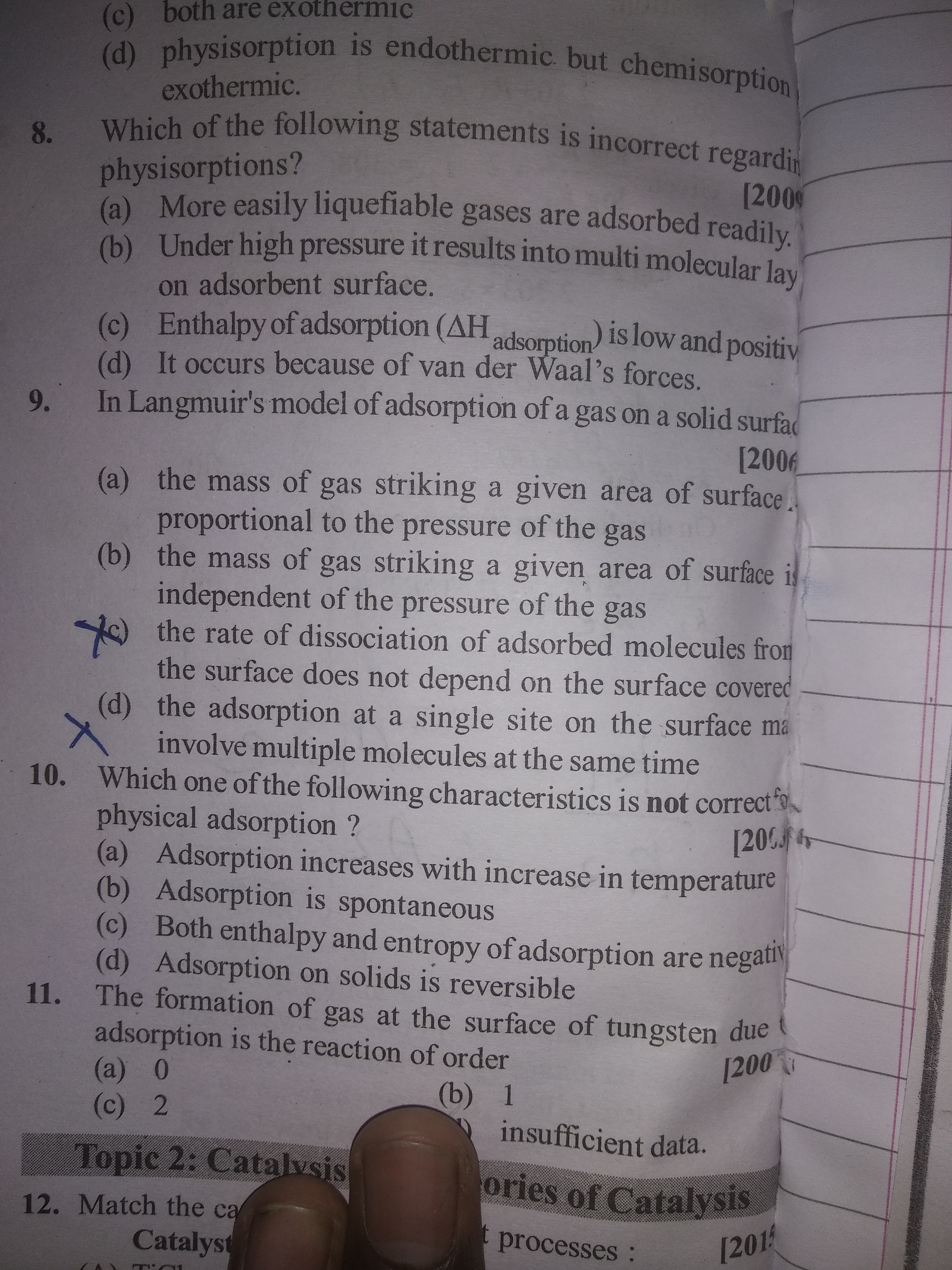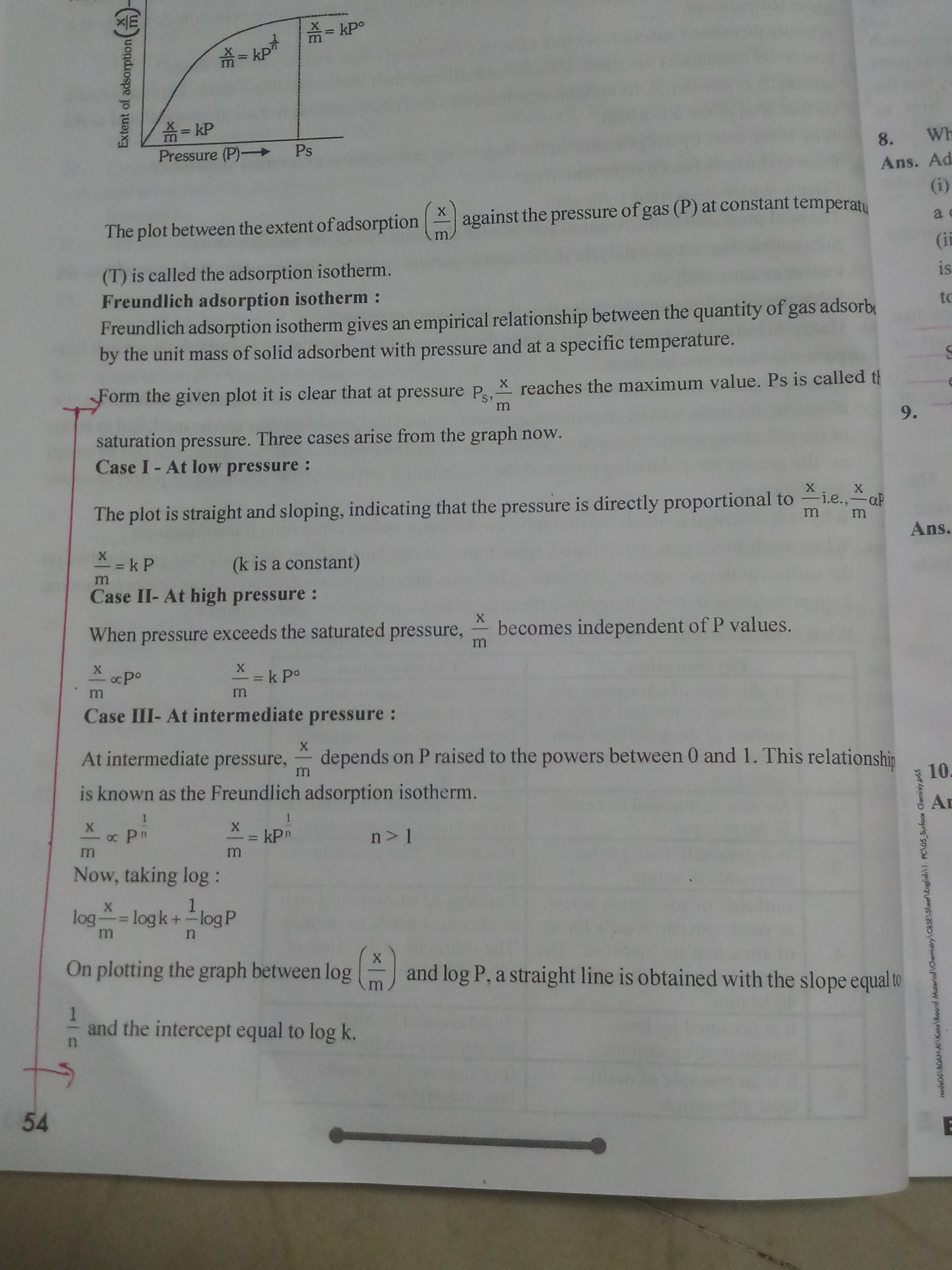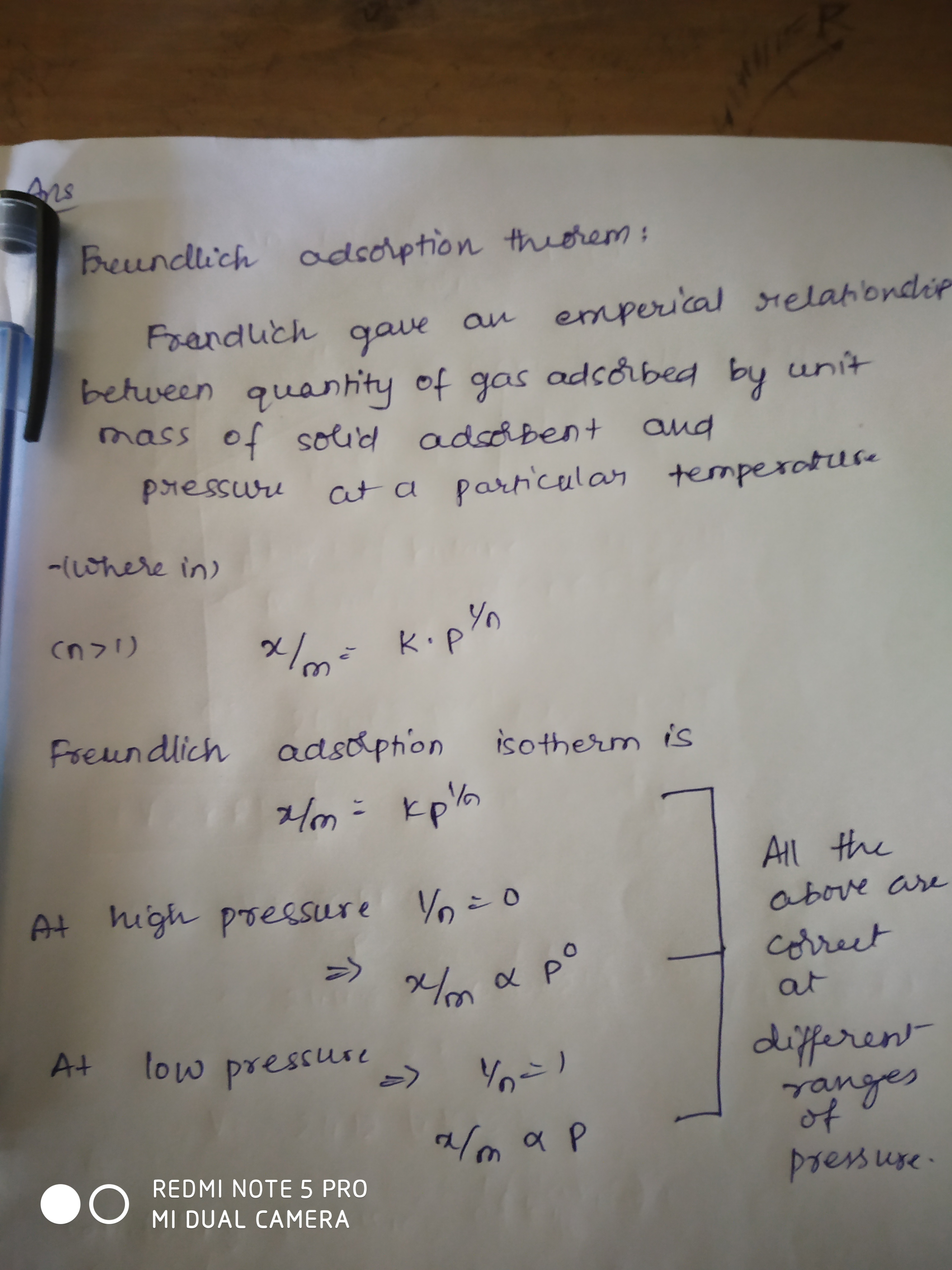Q5
Why b is correct asOur teacher have told is n is greater than 1 so how b can be correctQ9
But is a entirely correct as it says mass is directly proportional to pressure but do we say this relation a direct proportion(see below pic)For Freundlich's adsorption isotherm u can refer thisYour teacher has said n>1 considering the case of intermediate pressure

The relation of Langmuir adsorption is right. Note that mass is NOT directly proportional to pressure, it is only proportional as mentioned in option A

Does this mean if anything say y is a function of x does this mean y is propotional to x?

It depends upon function. Here on changing the value of pressure we get different amount of mass adsorbed so it can be said that it is proportional to pressure

Say for example, y=F(X)=constant. Even this can be said a function of x, but doesn't change its value on differing value of x . So it is a function of x but it's not proportional to x. Hope you understand this...1 Like

Thanks
Do you think only constant function is exception or any other function can be?

I don't think so....1 Like